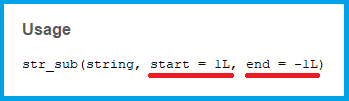# str_sub R Function of stringr Package (2 Examples)

This R programming tutorial explains how to apply the str_sub function of the stringr package. First, let’s have a look at the basic R syntax and the definition of the str_sub function:

Basic R Syntax of str_sub:

`str_sub(string = my_string, start = 3, end = 7)`

Definition of str_sub:

The str_sub function extracts or replaces a substring from a character string.

This article will show you two examples for the usage of str_sub in R.

Let’s dive in!

## Example 1: Extract Substring with str_sub

The first example shows how to extract a substring with the str_sub R function of the stringr package. Let’s first create an example character string in R:

`x <- "example_xxx_string"                                  # Create example string`

Before applying stringr functions such as str_sub to our example data, we also need to install and load the stringr package:

```install.packages("stringr")                                # Install stringr package

Now we can extract a substring from our example data as follows:

```str_sub(string = x, start = 8, end = 12)                   # Extract substring
# "_xxx_"```

As you can see based on the previous R code, we just had to specify:

• The string (i.e. x)
• The starting point (i.e. 8)
• The end point (i.e. 12)

Note that the start and end point are by default the first and last character of the input string. str_sub is therefore also useful, in case you want to truncate leading or trailing characters of a string. Further details can be found in the R help documentation of str_sub:Figure 1: Usage of str_sub Explained in the R Help Documentation.

## Example 2: Replace Substring with str_sub

The second example explains how to replace a substring with str_sub. We are again using our example string x, which we have created in Example 1.

We can now replace a substring of our example string with the following R code:

```str_sub(string = x, start = 8, end = 12) <- " character "  # Replace substring
x                                                          # Print updated string
# "example character string"```

Note what we have changed compared to Example 1: We just added an assignment arrow and the replacement at the end of the str_sub command (i.e. <- " character ").

## Tutorial Video & Further Resources of Dealing with Strings in R

If there are any questions left concerning the topic of this article, please check out the video below where I explain the content in detail:

Please accept YouTube cookies to play this video. By accepting you will be accessing content from YouTube, a service provided by an external third party.If you accept this notice, your choice will be saved and the page will refresh.

Handling characters in R can be a tricky task. For that reason, I have listed some further resources for the handling of character strings in the following. First, you might have a look at the following YouTube video of the channel expresstut01. In the video, the speaker is giving further examples for functions of the stringr package:

Please accept YouTube cookies to play this video. By accepting you will be accessing content from YouTube, a service provided by an external third party.If you accept this notice, your choice will be saved and the page will refresh.

Furthermore, I have already published several R tutorials on the handling of character strings on this website (including tutorials on other stringr functions):

In this tutorial, you have learned how to use the str_sub function in R. However, in case you have any further questions on the usage of str_sub, just leave me a comment below!

Subscribe to the Statistics Globe Newsletter